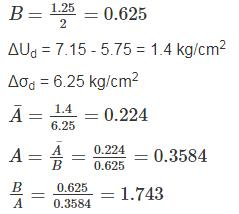Courses

# Test: Soil Mechanics- 6

## 10 Questions MCQ Test GATE Civil Engineering (CE) 2022 Mock Test Series | Test: Soil Mechanics- 6

Description
This mock test of Test: Soil Mechanics- 6 for Civil Engineering (CE) helps you for every Civil Engineering (CE) entrance exam. This contains 10 Multiple Choice Questions for Civil Engineering (CE) Test: Soil Mechanics- 6 (mcq) to study with solutions a complete question bank. The solved questions answers in this Test: Soil Mechanics- 6 quiz give you a good mix of easy questions and tough questions. Civil Engineering (CE) students definitely take this Test: Soil Mechanics- 6 exercise for a better result in the exam. You can find other Test: Soil Mechanics- 6 extra questions, long questions & short questions for Civil Engineering (CE) on EduRev as well by searching above.
QUESTION: 1

### The Contact pressure and settlement distribution for the footing are shown in the figure.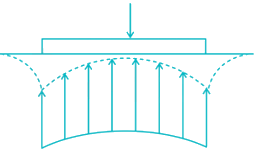The figure corresponds to a

Solution:

In flexible footing, settlement is non-uniform but contact pressure is more or less uniform. In case of sands, modulus of elasticity (E) increases with the confining pressure and therefore increases with the depth. Further ‘E’ is greater near the centre than at edges, As a result, the distribution of deformation below a flexible footing will be of the invested dish pattern while the contact pressure again be more or less uniform.

QUESTION: 2

### The stress-strain behavior of soils as shown in the figure corresponds to: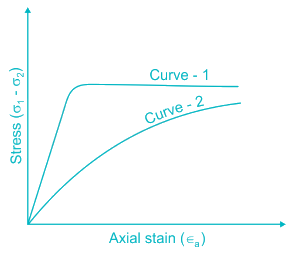Solution:

The stress – strain curves for dense sands and loose sands are similar in shape to the stress – strain curve for over consolidated and normally consolidated clays respectively.

QUESTION: 3

### Choose the incorrect statement: 1) Greater the confining pressure, smaller the critical void ratio. 2) A saturated loose sand is likely to liquify when subjected to earthquake shocks. 3) Failure envelope of over consolidated clay is a curve and not straight line. 4) For normally consolidated clays, ϕ decrease with an increase in plasticity.

Solution: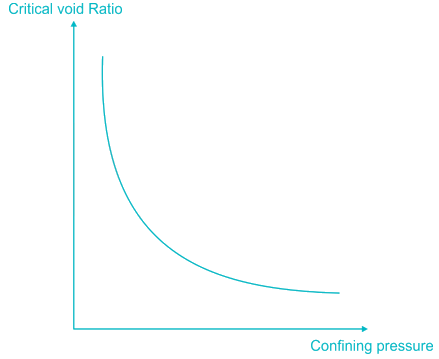The relationship between critical void ratio and confining pressure is shown in figure. As confining pressure increases, the critical void ratio decreases. With this knowledge of relationship it is possible predict the behaviour of sand at any ratio and at any given confining pressure.

All other statements are also true.

*Answer can only contain numeric values
QUESTION: 4

A sample of dry sand was subjected to tri - axial test with a confining pressure of 250 kN/m2. The angle of internal friction is found to be 36°. The sample is likely to fail at deviator stress of ________ kN/m2.

Solution:

Here, σ3 = 250 kN/m2

σ1 = σ3 + σd

Where, σd is the deviator stress

Using plastic equilibrium condition,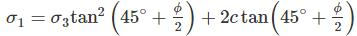As sandy soil, C = 0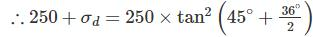⇒ 250 + σd = 962.96

⇒ σd = 712.95 kN/m2

QUESTION: 5

A multi-storied building is to be completed over a fine clayey soil within three months. In order to analyze the shear strength of the foundation soil which of the following shear strength test will be most suitable.

Solution:

The purpose of a UU test is to determine the undrained shear strength of a saturated soil. The UU test consists of applying a cell pressure to the soil sample without drainage of porewater followed by increments of axial stress. The cell pressure is kept constant and the test is completed very quickly because in neither of the two stages—consolidation and shearing—is the excess porewater pressure allowed to drain.
Suitability of UU test: When construction is very fast such as in case of foundation over fine clay.

QUESTION: 6

A cylindrical specimen of saturated clay, 3.5 cm in diameter and 8 cm in height were tested in an unconfined compression testing machine. The specimen fails under an axial load of 0.3 kN when the axial deformation was 8 mm. The unconfined compressive strength of the soil is

Solution: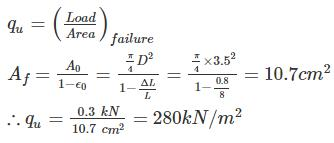QUESTION: 7

Stress path equation for tri - axial test upon application of deviatoric stress is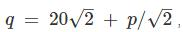the respective value of cohesion, C (kPa) and angle of internal friction is

Solution: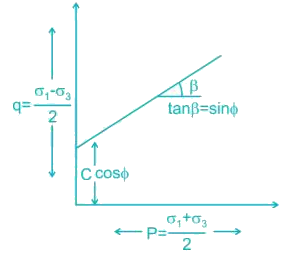Comparing the given equation with equation – (1)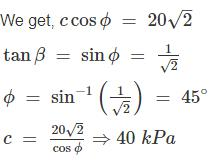*Answer can only contain numeric values
QUESTION: 8

​A commercial building circular in the plan is situated at a particular site. If the total load from the building including its self-weight is 150 kN/m2, determine the vertical stress (kN/m2) at a depth of 8 m below the C.G of the load area. Assume the diameter of the building to be 3 m.

Solution:

Concept:

Vertical pressure below uniformly loaded circular area is given by: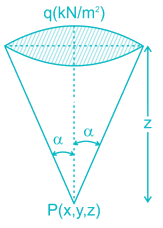σz = kq

where,

k = 1 – cos3 ∝

cos∝=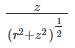Calculation:

q = 150 kN/m2

z = 8 m

r = 3/2m = 1.5m

cos∝ =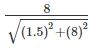cos ∝ = 0.983

k = 1 – cos3 ∝ = 1 – (0.983)3

k = 0.050

σz = 0.050 × (150)

σz = 7.5 kN/m2

*Answer can only contain numeric values
QUESTION: 9

A water tank is supported on 4 columns, which form a square of side 3m. The total load of the water tank is 400 ton. Assuming the load on each footing as point load, find the vertical stress induced at a depth of 3m under any one of the footings? (In t/m2)

Solution:

The arrangement of the column / footing will be as follows: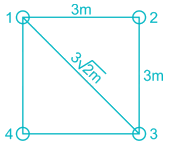load in each column, Q = 400/4 = 100 ton
∴ Stress at a depth of 3m under any column

(Two column will be at a distance 3 m & one column will be at a distance 3√2m)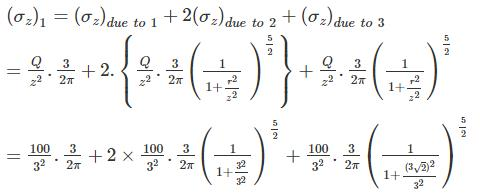= 5.30+ 1.876+ 0.34

= 7.516 ton/m2

*Answer can only contain numeric values
QUESTION: 10

A soil sample was consolidated under a cell pressure of 8 kg/cm2, pore water pressure of 4.5 kg/cm2. With no drainage permitted the cell pressure was raised to 10 kg/cm2 results in increased pore water pressure to 5.75 kg/cm2. The axial load was then increased to give a deviator stress of 6.25 kg/cm2 (with the cell pressure at 10 kg/cm2), pore pressure is now noted to be 7.15 kg/cm2. The ratio of pore pressure coefficients B to A is ______.

Solution:

Concept:

Pure pressure parameter (B)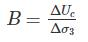σ= cell pressure

U= pore pressure

Δσ3 = change in cell pressure in cell pressure stage

ΔUC = change in pore pressure in cell pressure stage

Pore pressure parameter (A)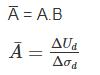ΔUd = change in pore in deviator state

Δσd = change in deviator stress

Calculation:

ΔUc = 5.75 - 4.5 = 1.25 kg/cm2

Δσ3 = 10 - 8 = 2 kg/cm2